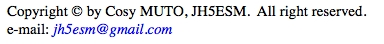Cosy MUTO, JH5ESM
Written in Japanese on 29 Dec., 2005
English translated on 30 Dec., 2005

## 1. Introduction

The method is quite simple and useful in actual design.

The concept of Smith chart is out of the range from this document, so the readers not familiar with this should study it elsewhere in the world :p

## 2. A design example

Consider a horizontal 3.5/10MHz element shown in Fig.1.  It is a monopole element so that an opposite element is required for a dipole realization.

L1, L2 and LH represent 10MHz λ/4 element, 3.5MHz element and 10MHz adjuster, respectively.In this example, L1 and L2 length are 7.42[m] and 4.2[m], respectively.  The latter comes from my antenna space limitation.

Since the elements L1 and L2 have already been determnied, plot these electrical length at 3.5MHz on the Smith chart.

L1 is 0.087λ at 3.5MHz, so plot a counter clockwise arc from 0[Ω] to 0.087λ position as shown in Fig.2.
L2 is 0.049λ at 3.5MHz, so plot a clockwise arc from ∞[Ω] (open end) by 0.049λ.The reactance of 0.087λ element from the feeding point is 0.61Z0, and that of 0.049λ element from open end is 3.15Z0.
Therefore, the reactance of the loading inductor becomes approximately 2.6Z0, where Z0 designates characteristics impedance of the element wire.

Let Z0 be 500[Ω], the loading inductor is then determined as 59[μH].

## 4. Adjuster length at higher band

The element L1 itself resonates on higher frequency band and the loading inductor and lower band element L2 are redundant on that band.  The aim of the adjuster is to cancel out the loading inductor and lower band element at the higher band.Plot a clockwise arc from ∞[Ω] (open end) by the electrical length of L2 at higer frequency band.  In this case, 4.2[m] element corresponds to 0.141λ at 10MHz band (green arc plot in Fig.3).

Next, calculate the loading inductor reactance at higher frequency band and plot a clockwise arc from L2 reactance position by that value (blue arc plot in Fig.3). The resultant position implies the impedance seen from the far end of higher band element.

The adjuster length LH can be easily obtained by the electrical length from the above mentioned point to ∞ [Ω] point (red arc plot in Fig.3).

That's the end of the design.

## 5. Resources

The antenna tuning is made by the following procedures:

1. Disconnect higher band element (L1) and all the other component.
2. Tune to the higher band.
4. Tune to the higher band again by the adjuster LH.

### 5.2 The Smith chart resources on the web

The Smith Chart page on Spread Spectrum Scene has a lot of information on Smith chart.
"A Great Smith Chart -- 264k .zip file format" file, at the "Some Smith Chart Examples" section on that page is a 300 dpi GIF format Smith chart.

### 5.3 Characteristic impedance of horizontal single wire element

Characteristic impedance of a horizontal single wire (Fig.4) is determined by the following equation;

Z0 = 138 log10 (4h/d)  [Ω]

Figure 5 show some calculation results for specific wire size and height.Fig.4 A horizontal single wire above the ground.Fig.5 Characteristic impedance of a horizontal single wire.